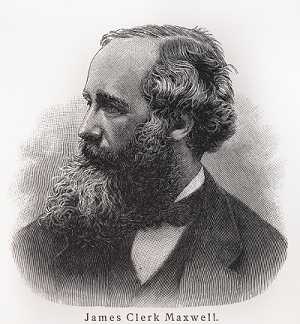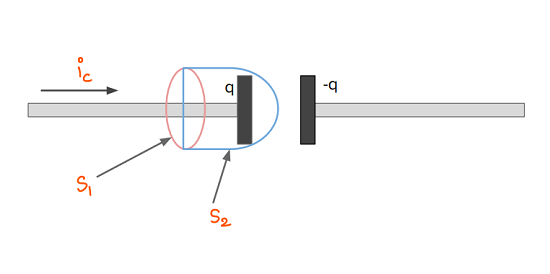# Displacement Current

## Introduction

Initially, electricity and magnetism were treated as separate subjects. In a later period due to the contribution of Oersted, Faraday, Maxwell, etc., it evolved as a unified subject.The current-carrying wire creates a magnetic field around it. Faraday through his experiments showed that current can be produced even if there are no batteries in the circuit. The change in the magnetic field can produce a current in the circuit. This result is known as electromagnetic induction. Maxwell tried to write all the main equations of electricity and magnetism in a unified and compact manner. These equations are known as maxwell’s equations.

This set of equations contains - gauss’s theorem of electrostatic, Gauss’ law for magnetism, Ampere’s circuital law, and Faraday’s laws of Electromagnetic induction. Maxwell found some inconsistencies in Ampere’s law. He modified this equation and introduced a new concept called displacement current. Therefore this equation is sometimes also known as the Maxwell-ampere equation.

## What is Displacement Current?

James clerk Maxwell introduced this concept. If there is a region where charges are flowing and the electric current is present, then the magnetic field arises due to it. According to Maxwell, in space, the magnetic field arises due to displacement current. Unlike the conventional current, it is not composed of electric charges. Since there is a free space between the plates of the capacitor. We can derive the expression of displacement current by taking the example of a capacitor.

## Displacement Current Equation

To arrive at the displacement current equation, we use the example of a capacitor. We try to find the magnetic field using the ampere’s law -

$$\mathrm{\oint \overrightarrow{B}.\overrightarrow{dl}=\mu _{0}i_{enc}}$$

Where B = Magnetic field, i = net current passing through the surface.First, we try to calculate the magnetic field by taking surface $\mathrm{S_{1}}$. The current passing through this surface is $\mathrm{i_{c}}$. Hence there will be some magnetic field according to ampere’s law. If we take surface $\mathrm{S_{2}}$ then there will be no current passing through the surface. This means $\mathrm{\overrightarrow{B}.\overrightarrow{dl}}$𝑙zero and non-zero at the same time. It is a contradictory result.

Maxwell solved this problem by introducing a displacement current.

$$\mathrm{i_{d}=\epsilon _{0}\frac{d \phi _{E}}{dt}}$$

Here $\mathrm{i_{d}}$= Displacement current

$\mathrm{\phi _{E}}$ = Electric Flux

We can include this term in ampere’s law.

$$\mathrm{\oint \overrightarrow{B}.\overrightarrow{dl}=\mu _{0}(i_{c}+i_{d})=\mu _{0}i_{c}+\mu _{0}\epsilon _{0}\frac{d\phi _{E}}{dt}}$$

It is known as the Maxwell-ampere equation.

If we use this relation, we can resolve the ambiguity arising in the capacitor problem.

For surface $\mathrm{S_{1}:i=i_{c}}$ and $\mathrm{i_{d}=0}$ since there is no change is electric flux.

For surface $\mathrm{S_{2}:i=i_{d}}$ and $\mathrm{i_{c}=0}$ since there are no charges.

Hence, Now the magnetic field will be non-zero for both surfaces.

## Characteristics of Displacement Current

The displacement current is produced due to varying electric flux. It can be written as -

$$\mathrm{i_{d}=\epsilon _{0}\frac{d\phi _{E}}{dt}}$$

It has the following characteristics -

• It is a vector quantity.

• It is measured in Amperes and its dimension are similar to the conventional current.

• It is not produced by the flow of electrons but by the change in the electric field.

• It doesn’t follow ohm’s law.

• For a closed path, it is zero.

• It is not an actual current.

## Application of Displacement Current

We can calculate the magnetic field inside a circular capacitor using displacement current.

Suppose the radius of capacitor plates is R, then we imagine a circular wire of radius R between these plates. We take the current flowing in the wire equal to the displacement current $\mathrm{i_{d}}$. We can use the ampere’s circuital law here. Since there is only displacement current between the plates -

$$\mathrm{\oint \overrightarrow{B}.\overrightarrow{dl}=B\oint dl=B2\pi r}$$

r= field point inside the capacitor from the centre.

The electric current is uniformly distributed and the current enclosed by the loop $\mathrm{i_{enc}}$, is proportional to the area enclosed by the loop. Hence

$$\mathrm{i_{enc}=i_{d}\frac{\pi r^{2}}{\pi R^{2}}}$$

This means

$$\mathrm{B2\pi r=\mu _{0}i_{enc}}$$

$$\mathrm{B2\pi r=\mu _{0}i_{d}\frac{\pi r^{2}}{\pi R^{2}}}$$

$$\mathrm{B=(\frac{\mu _{0}i_{d}}{2\pi R^{2}})r}$$

It is the magnetic field inside the capacitor. It is inversely proportional to the square of the radius of capacitor plates and directly proportional to the distance between the field point from the center.

We can also calculate the magnetic field outside the circular capacitor, it would be -

$$\mathrm{B=\frac{\mu _{0}i_{d}}{2\pi r}}$$

## Conclusion

Maxwell found some inconsistencies in the ampere’s law. He modified this equation by introducing a current known as displacement current. Displacement current doesn’t arise from the flow of electric charges but the varying electric flux. Its units and dimension are the same as conventional current.

## FAQs

Q1. Write down Maxwell’s equations of Electromagnetism.

Ans. Maxwell’s equation of electromagnetism are following -

• $\mathrm{\oint \overrightarrow{E}.\overrightarrow{dA}=\frac{q_{enc}}{\epsilon _{0}}}$ ,It relates net charge to the electric flux.

• $\mathrm{\oint \overrightarrow{E}.\overrightarrow{dA}=0}$,It relates net magnetic charge to magnetic flux.

• $\mathrm{\oint \overrightarrow{E}.\overrightarrow{dA}=-\frac{d\phi _{B}}{dt}}$ ,It relates change in magnetic flux to the induced electric field.

• $\mathrm{\oint \overrightarrow{B}.\overrightarrow{dl}=\mu _{0}i_{c}+\mu _{0}\epsilon _{0}\frac{d\phi _{E}}{dt}}$ ,It relates induced magnetic field to the current.

Q2. A circular capacitor of a radius of 2cm, has a displacement current of 4A, Find the magnetic field at a distance R/4 inside the capacitor.

Ans. It is given that the R = 2cm = $\mathrm{2\times 10^{-2}m}$

$$\mathrm{I = 4A}$$

$$\mathrm{r=\frac{R}{4}}$$

We can use the formula which we derived in the previous section -

$$\mathrm{B=(\frac{\mu _{0}i_{d}}{2\pi R^{2}})r}$$

$$\mathrm{B=\frac{4\pi \times 10^{-7}\times 4}{2\pi \times R^{2}}\frac{R}{4}=\frac{2\times 10^{-7}\times 4}{R\times 4}}$$

$$\mathrm{B=\frac{2\times 10^{-7}}{2 \times 10^{-2}}=10^{-5}}$$

$$\mathrm{B=10^{-5}T}$$

Q3. State the Ampere’s circuital law.

Ans. Ampere’s law can be stated in the following way - “ The line integral of the force of field throughout any closed coil containing the steady current is proportional to the current passing through that coil.”

Suppose there is a closed path C and the net current passing through it is $\mathrm{i_{enc}}$. Then we can write

$$\mathrm{\oint \overrightarrow{B}.\overrightarrow{dl}=\mu _{0}i_{enc}}$$

Q4. A parallel plate capacitor at a potential of 100 V. If the distance between the plates of $\mathrm{}$𝟐. Calculate the displacement current for $\mathrm{}$.

Ans. The potential of parallel plate capacitor V = 100V

Distance between the plates d = 2mm = $\mathrm{2\times 10^{-3}mm}$

$$\mathrm{Area\: of\: plates\: A\:=\:20\times 10^{-4}m^{2}}$$

$$\mathrm{Time\:t=2\mu s=2\times 10^{-6}s}$$

$$\mathrm{Displacement\: current\:i_{d}=\epsilon _{0}\frac{d\phi }{dt}=\epsilon _{0}\frac{EA}{t}}$$

We know that $\mathrm{E=\frac{v}{d} }$

$$\mathrm{Hence,i_{d}=\epsilon _{0}\frac{vA }{dt}=8.85\times 10^{-12}\frac{10^{2}\times 20\times 10^{-4}}{2\times 10^{-3}\times 10^{-6}\times 2 }}$$

$$\mathrm{i_{d}=\frac{8.85\times 5\times 10^{-16}}{10^{-9}}=8.85\times 5\times 10^{-7}}$$

$$\mathrm{i_{d}=44.25\times 10^{-7}A}$$

$$\mathrm{i_{d}=4.4\mu A}$$

Q5. Is it necessary to change of electric field for the existence of displacement current?

Ans. The displacement current can exist if there is a change in electric flux. It may be possible in some cases where the electric field is not changing but overall flux is varying.